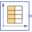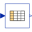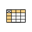# Smoothness

Enumeration defining the smoothness of table interpolation

# Enumeration Literals (5)

LinearSegments Table points are linearly interpolated Table points are interpolated (by Akima splines) such that the first derivative is continuous Table points are not interpolated, but the value from the previous abscissa point is returned Table points are interpolated (by Fritsch-Butland splines) such that the monotonicity is preserved and the first derivative is continuous Table points are interpolated (by Steffen splines) such that the monotonicity is preserved and the first derivative is continuous

# Used in Components (4)CombiTimeTable Modelica.Blocks.Sources Table look-up with respect to time and linear/periodic extrapolation methods (data from matrix/file)CombiTable1D Modelica.Blocks.Tables Table look-up in one dimension (matrix/file) with n inputs and n outputsCombiTable1Ds Modelica.Blocks.Tables Table look-up in one dimension (matrix/file) with one input and n outputsCombiTable2DBase Modelica.Blocks.Tables.Internal Base class for variants of CombiTable2D